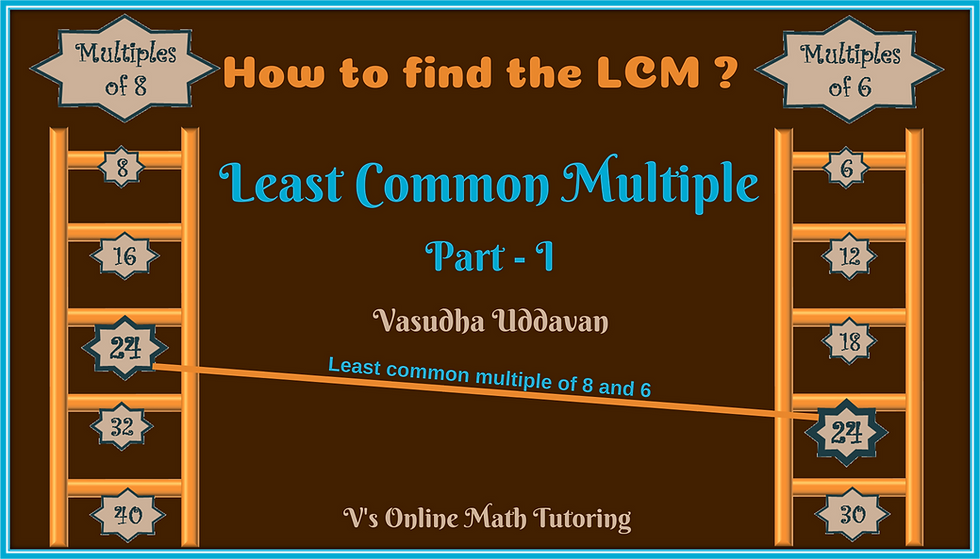top of pageSearch

# How to find the LCM (Least Common Multiple) and how I teach it

Updated: Nov 29, 2018

I love teaching GCFs and LCMs to kids. My latest craze in Mathematics is Number Theory. Wiki defines Number theory (or arithmetic or higher arithmetic in older usage) as a branch of pure mathematics devoted primarily to the study of the integers. So in layman's terms the basics of number theory deals with the numbers that we use in day to day life. When I teach these to kids, they get more comfortable in seeing the relationships that numbers have with each other. They become faster and more comfortable in arithmetic.How to find the LCM (Least Common Multiple) of 2 numbers

So how do I teach them how to find LCM? Here goes:

First Method: Listing all the Multiples

Example 1 - Let's find the LCM of 6 and 8

List all the multiples of 6 and 8. Multiples are basically all numbers that comes in that number's (in this case 6's and 8's) multiplication table.

Multiples of 6: 6, 12, 18, 24, 30, 36, 42, 48, 54, 60, 66, 72 ......

Multiples of 8: 8, 16, 24, 32, 40, 48, 56, 64, 72 ....

Pick out all the common multiples - 24, 48, 72 ....

The least among them is 24. Hence the LCM is 24How to find the LCM of 6 and 8

Example 2 - Find the LCM of 3 and 5

So once again list all the multiples of 3 and 5

Multiples of 3: 3, 6, 9, 12, 15, 18, 21, 24, 27, 30, 33 ....

Multiples of 5: 5, 10 ,15, 20, 25, 30, 35, 40 .....

The Common Multiples are: 15, 30 ....

The least among them is 15. Hence the LCM of 3 and 5 is 15

Example 3: Find the LCM of 8 and 9

Again list all the multiples of 8 and 9

Multiples of 8: 8, 16, 24, 32, 40, 48, 56, 64, 72, 80 .....

Multiples of 9: 9, 18, 27, 36, 45, 54, 63, 72, 81, 90 ......

Common multiples that we can see: 72. Hence the LCM is 72.

A question: What do you notice among all the common multiples?

Go to the bottom of this post for the answer.

Now can you find the LCM of 13 and 15?

If you start listing all the multiples do you see how long it takes?

What happens if I give larger numbers like 24 and 30? Is there a more efficient way of doing this? Of course there is a more efficient way. We can use the L-method. We'll see that in Part 2 of this series.

But a few things to note before we finish this method.

The kids have been finding LCM even before it has been taught formally in school. Where is it used? In adding or subtracting fractions with Unlike Denominators.

How do you add 2/5 + 1/3?

We make them into equivalent fractions.

6/15 + 5/15 = 11/15

Do you see that we've decided that the denominator has to be common and we chose 15? Because that's the LCM of 3 and 5.

So I also guess that this answer the most popular question - Why do we need LCM and where do we use it :)

And finally before we end the answer to - What do you notice among all the common multiples?

Answer: They are all multiples of the LCM.

The LCM of 6&8 is 24. And all the common multiples are 24, 48, 72 an so on.

The LCM of 3&5 is 15. And all the common multiples are 15 ,30 and as you can guess the next common multiple is going to be 45.

The LCM of 8&9 is 72. Can you guess the next 3 common multiples?

Why is this info important? To be able to solve problems like these in math competitions.

Problem: Find a number between 300 and 400 that is a multiple of both 8 and 9.Interesting Math Problems - Problems that make you think :)

Use the hint above to figure it out and Post your answers in the comments section below.

Related Posts:

How to find the LCM and how I teach it - Part 2How to find the LCM by Ladder Method